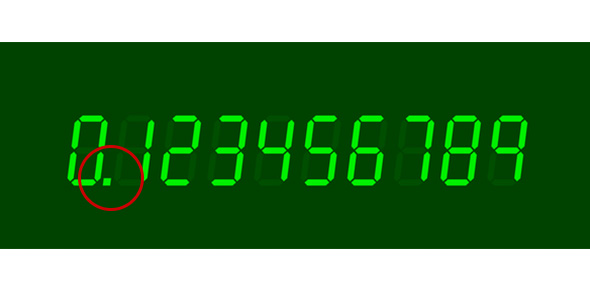# Math 090 Decimals Quiz

9 Questions | Attempts: 357SettingsThis is a quiz on decimals

• 1.
What is 2.5 X .88?
• A.

2.2

• B.

2.67

• C.

None of these

• 2.
What is 5.25 divided by .05?
• A.

10.5

• B.

105

• C.

None of these

• 3.
Convert 5/8 into a decimal.
• A.

.685

• B.

5.8

• C.

None of these

• 4.
What is 13 - 6.87?
• A.

0.613

• B.

613

• C.

None of these

• 5.
Convert 5 3/5 into a decimal.
• A.

0.6

• B.

5.6

• C.

None of these

• 6.
To add or subtract decimals, you must line up the decimal points.
• A.

True

• B.

False

• C.

Depends

• 7.
Multiply (1.3)(2.4)(6.8)
• A.

21.216

• B.

212.16

• C.

None of these

• 8.
100 - .999
• A.

1

• B.

99

• C.

None of these

• 9.
Round 34.657 to the nearest tenths place.
• A.

34.7

• B.

34.66

• C.

None of these

## Related TopicsBack to top
×

Wait!
Here's an interesting quiz for you.